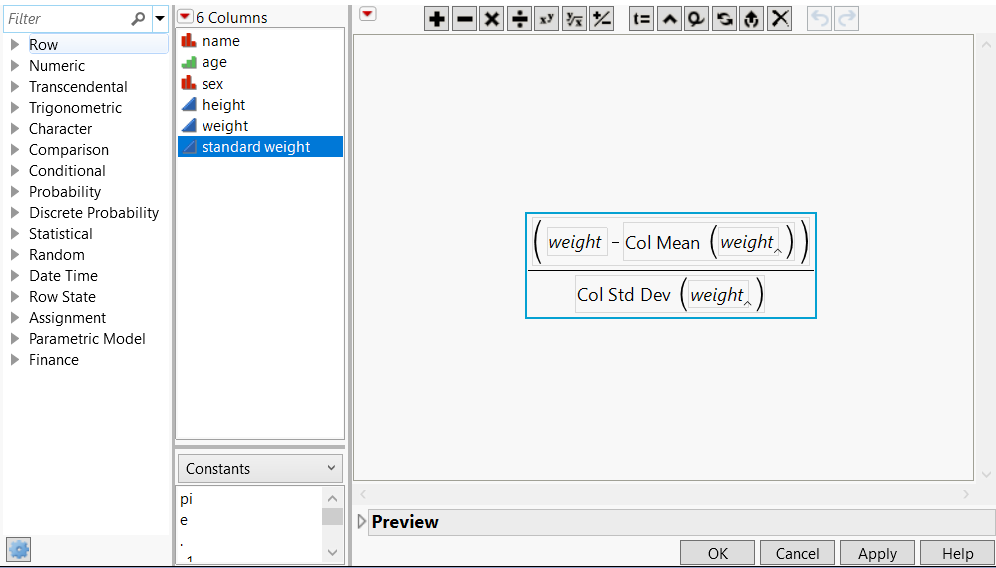Using JMP > Formula Editor
Publication date: 07/30/2020

# Formula Editor

##### Construct Formulas

Use the JMP Formula Editor to create a column whose values are computed by a formula and store that formula as part of a column’s information. Formulas can be simple assignments of numeric, character, or row state constants, or they can contain complex evaluations based on conditional clauses. The Formula Editor window operates like a calculator with buttons, displays, and a list of functions.

For more information about the functions that are available in the Formula Editor, see Formula Functions Reference.

Figure 7.1 The Formula Editor